CBSE Class 12 Sample Paper for 2021 Boards

Class 12
Solutions of Sample Papers and Past Year Papers - for Class 12 Boards

## Check whether the relation R in the set Z of integers defined as R = {(𝑎, 𝑏) ∶ 𝑎 + 𝑏 is "divisible by 2"} is reflexive, symmetric or transitive. Write the equivalence class containing 0 i.e. .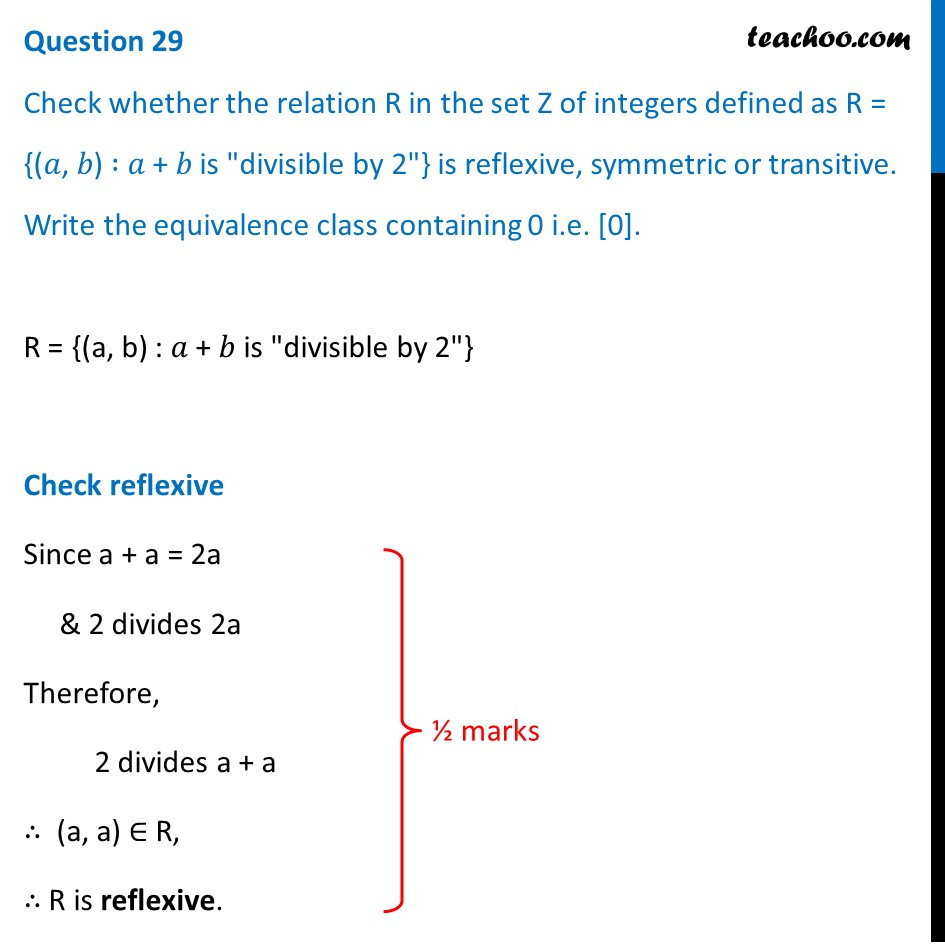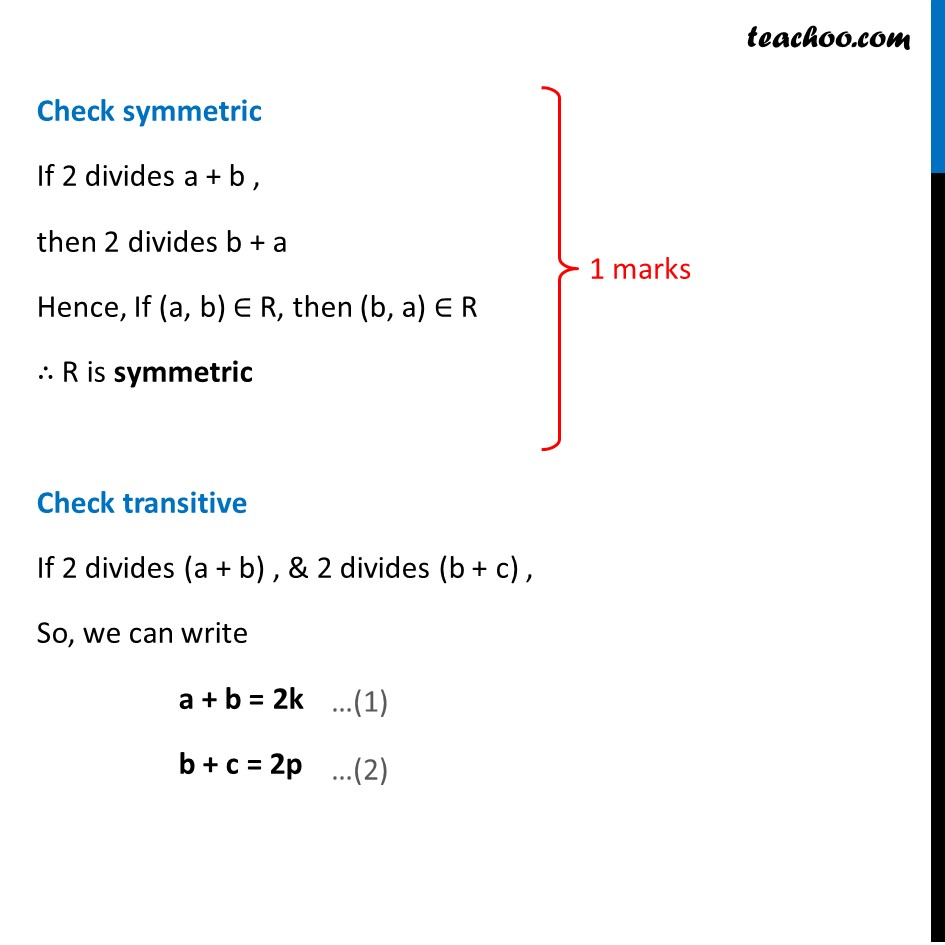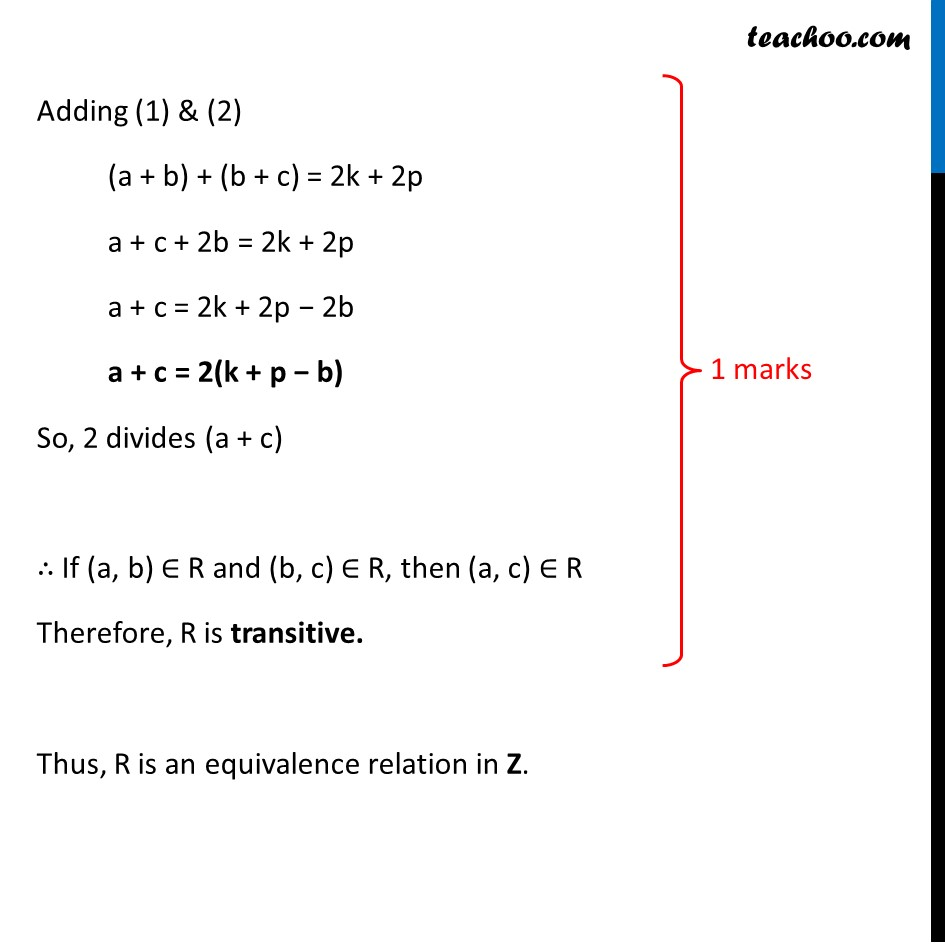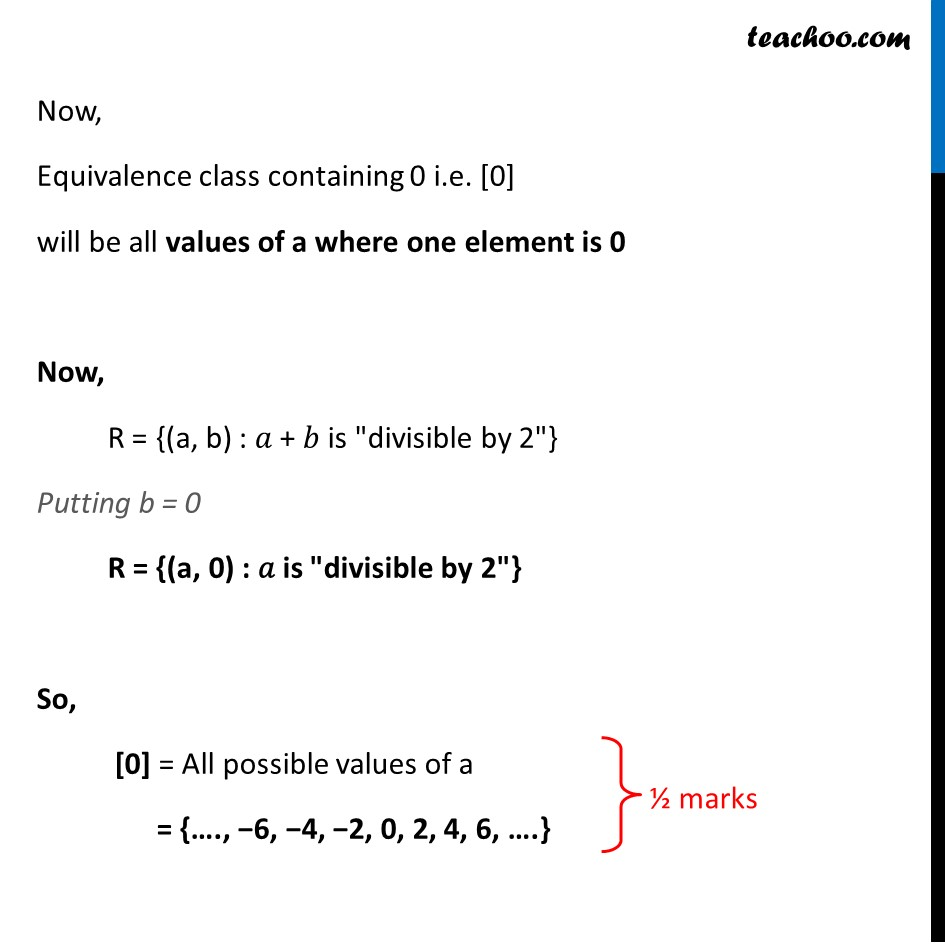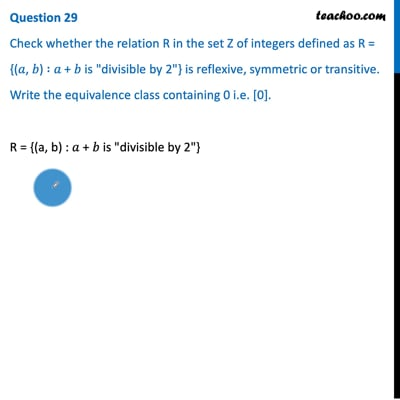This video is only available for Teachoo black users

Note : This is similar to Example 5 of NCERT – Chapter 1 Class 12 Relations and Functions

Introducing your new favourite teacher - Teachoo Black, at only ₹83 per month

### Transcript

Question 29 Check whether the relation R in the set Z of integers defined as R = {(𝑎, 𝑏) ∶ 𝑎 + 𝑏 is "divisible by 2"} is reflexive, symmetric or transitive. Write the equivalence class containing 0 i.e. . R = {(a, b) : 𝑎 + 𝑏 is "divisible by 2"} Check reflexive Since a + a = 2a & 2 divides 2a Therefore, 2 divides a + a ∴ (a, a) ∈ R, ∴ R is reflexive. Check symmetric If 2 divides a + b , then 2 divides b + a Hence, If (a, b) ∈ R, then (b, a) ∈ R ∴ R is symmetric Check transitive If 2 divides (a + b) , & 2 divides (b + c) , So, we can write a + b = 2k b + c = 2p Adding (1) & (2) (a + b) + (b + c) = 2k + 2p a + c + 2b = 2k + 2p a + c = 2k + 2p − 2b a + c = 2(k + p − b) So, 2 divides (a + c) ∴ If (a, b) ∈ R and (b, c) ∈ R, then (a, c) ∈ R Therefore, R is transitive. Thus, R is an equivalence relation in Z. Now, Equivalence class containing 0 i.e.  will be all values of a where one element is 0 Now, R = {(a, b) : 𝑎 + 𝑏 is "divisible by 2"} Putting b = 0 R = {(a, 0) : 𝑎 is "divisible by 2"} So,  = All possible values of a = {…., −6, −4, −2, 0, 2, 4, 6, ….}